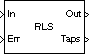Compute filter estimates for input using RLS adaptive filter algorithm

## Library

`dspobslib`

•## Description

Note

The RLS Adaptive Filter block is still supported but is likely to be obsoleted in a future release. We strongly recommend replacing this block with the RLS Filter block.

The RLS Adaptive Filter block recursively computes the recursive least squares (RLS) estimate of the FIR filter coefficients.

The corresponding RLS filter is expressed in matrix form as

`$\begin{array}{l}k\left(n\right)=\frac{{\lambda }^{-1}P\left(n-1\right)u\left(n\right)}{1+{\lambda }^{-1}{u}^{H}\left(n\right)P\left(n-1\right)u\left(n\right)}\hfill \\ y\left(n\right)={\stackrel{^}{w}}^{H}\left(n-1\right)u\left(n\right)\hfill \\ e\left(n\right)=d\left(n\right)-y\left(n\right)\hfill \\ \stackrel{^}{w}\left(n\right)=\stackrel{^}{w}\left(n-1\right)+k\left(n\right){e}^{*}\left(n\right)\hfill \\ P\left(n\right)={\lambda }^{-1}P\left(n-1\right)-{\lambda }^{-1}k\left(n\right){u}^{H}\left(n\right)P\left(n-1\right)\hfill \end{array}$`

where λ-1 denotes the reciprocal of the exponential weighting factor. The variables are as follows

VariableDescription

n

The current algorithm iteration

u(n)

The buffered input samples at step n

P(n)

The inverse correlation matrix at step n

k(n)

The gain vector at step n

$\stackrel{^}{w}\left(n\right)$

The vector of filter-tap estimates at step n

y(n)

The filtered output at step n

e(n)

The estimation error at step n

d(n)

The desired response at step n

λ

The exponential memory weighting factor

The block icon has port labels corresponding to the inputs and outputs of the RLS algorithm. Note that inputs to the `In` and `Err` ports must be sample-based scalars. The signal at the `Out` port is a scalar, while the signal at the `Taps` port is a sample-based vector.

Block PortsCorresponding Variables
`In`

u, the scalar input, which is internally buffered into the vector u(n)

`Out`

y(n), the filtered scalar output

`Err`

e(n), the scalar estimation error

`Taps`

$\stackrel{^}{w}\left(0\right)$, the vector of filter-tap estimates

An optional `Adapt` input port is added when you select the Adapt input check box in the dialog box. When this port is enabled, the block continuously adapts the filter coefficients while the `Adapt` input is nonzero. A zero-valued input to the `Adapt` port causes the block to stop adapting, and to hold the filter coefficients at their current values until the next nonzero `Adapt` input.

The implementation of the algorithm in the block is optimized by exploiting the symmetry of the inverse correlation matrix P(n). This decreases the total number of computations by a factor of two.

The FIR filter length parameter specifies the length of the filter that the RLS algorithm estimates. The Memory weighting factor corresponds to λ in the equations, and specifies how quickly the filter “forgets” past sample information. Setting λ=`1` specifies an infinite memory; typically, `0.95`≤λ≤`1`.

The Initial value of filter taps specifies the initial value $\stackrel{^}{w}\left(0\right)$ as a vector, or as a scalar to be repeated for all vector elements. The initial value of P(n) is

`$I\frac{1}{{\stackrel{^}{\sigma }}^{2}}$`

where you specify${\stackrel{^}{\sigma }}^{2}$ in the Initial input variance estimate parameter.

## Examples

The `rlsdemo` example illustrates a noise cancellation system built around the RLS Adaptive Filter block.

## Parameters

FIR filter length

The length of the FIR filter.

Memory weighting factor

The exponential weighting factor, in the range `[0,1]`. A value of `1` specifies an infinite memory. Tunable (Simulink).

Initial value of filter taps

The initial FIR filter coefficients.

Initial input variance estimate

The initial value of 1/P(n).

Enables the `Adapt` port.

## References

Haykin, S. Adaptive Filter Theory. 3rd ed. Englewood Cliffs, NJ: Prentice Hall, 1996.

## Supported Data Types

• Double-precision floating point

• Single-precision floating point